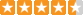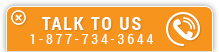Gas Pipeline Hydraulics

Course Number: O-5001
Credit: 5 PDH
Subject Matter Expert: E. Shashi Menon, P.E.
Price: \$149.75 Purchase using Reward Tokens. Details46 reviews
Overview

In Gas Pipeline Hydraulics , you'll learn ...

• Gas properties overview, including viscosity, pressure, compressibility factor and gas laws
• How to calculate the properties of a natural gas mixture
• How to calculate the compressibility factor of a gas using the Standing-Katz chart
• Friction factor and transmission factors used in gas pipeline pressure drop equations

OverviewPreview a portion of this course before purchasing it.

Credit: 5 PDH

Length: 59 pages

This online course on gas pipeline hydraulics covers the steady state analysis of compressible fluid flow through pipelines. Mathematical derivations are reduced to a minimum, since the intent is to provide the practicing engineer a practical tool to understand and apply the concepts of gas flow in pipes. In particular, we will cover natural gas pipeline transportation including how pipelines are sized for a particular flow rate, the pressure required to transport a given volume of gas and the compression horsepower required.

The properties of natural gas that affect pipe flow will be reviewed first followed by the concepts of laminar and turbulent flow and Reynolds number. Frictional pressure loss and the method of calculating the friction factor using the Moody diagram and the Colebrook and AGA methods will be illustrated with examples. Several other popular flow equations, such as the Weymouth and Panhandle formulas will be introduced and explained with example problems. Increasing pipeline throughput using intermediate compressor stations as well as pipe loops will be discussed. The strength requirement of pipes, allowable operating pressure and hydrostatic test pressure will be reviewed with reference to the DOT code requirements. Several fully solved example problems are used to illustrate the concepts introduced in the various sections of the course.

Specific Knowledge or Skill Obtained

This course teaches the following specific knowledge and skills:

• Gas properties overview, including viscosity, pressure, compressibility factor and gas laws
• How to calculate the properties of a natural gas mixture
• How to calculate the compressibility factor of a gas using the Standing-Katz chart
• Friction factor and transmission factors used in gas pipeline pressure drop equations
• Calculating pressure drop due to friction in a gas pipeline using the General Flow Equation
• Gas pipeline velocity and erosional velocity calculations
• Use of other gas pipeline pressure drop equations, including Panhandle A, Panhandle B, Weymouth and the IGT equation
• Compressor station fundamental calculations, including compression ratio, efficiency and horsepower
• Calculation of allowable pipe pressure using design factors based on the location of the pipeline and its proximity to populated areas
• The use of compressor stations and pipeline looping to increase gas throughput

Certificate of Completion

You will be able to immediately print a certificate of completion after passing a multiple-choice quiz consisting of 25 questions. PDH credits are not awarded until the course is completed and quiz is passed.

Board Acceptance
 This course is applicable to professional engineers in: Alabama (P.E.) Alaska (P.E.) Arkansas (P.E.) Delaware (P.E.) Florida (P.E. Area of Practice) Georgia (P.E.) Idaho (P.E.) Illinois (P.E.) Illinois (S.E.) Indiana (P.E.) Iowa (P.E.) Kansas (P.E.) Kentucky (P.E.) Louisiana (P.E.) Maine (P.E.) Maryland (P.E.) Michigan (P.E.) Minnesota (P.E.) Mississippi (P.E.) Missouri (P.E.) Montana (P.E.) Nebraska (P.E.) Nevada (P.E.) New Hampshire (P.E.) New Jersey (P.E.) New Mexico (P.E.) New York (P.E.) North Carolina (P.E.) North Dakota (P.E.) Ohio (P.E. Self-Paced) Oklahoma (P.E.) Oregon (P.E.) Pennsylvania (P.E.) South Carolina (P.E.) South Dakota (P.E.) Tennessee (P.E.) Texas (P.E.) Utah (P.E.) Vermont (P.E.) Virginia (P.E.) West Virginia (P.E.) Wisconsin (P.E.) Wyoming (P.E.)
Reviews (46)
More DetailsPreview a portion of this course before purchasing it.

Credit: 5 PDH

Length: 59 pages